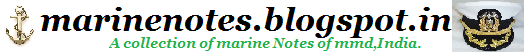Home » » Net Positive Suction Head

-->

To avoid cavitation in centrifugal pumps, the pressure of the fluid at all points within the pump
must remain above saturation pressure. The quantity used to determine if the pressure of the
liquid being pumped is adequate to avoid cavitation is the net positive suction head (NPSH).
The net positive suction head available (NPSHA) is the difference between the pressure at the
suction of the pump and the saturation pressure for the liquid being pumped. The net positive
suction head required (NPSHR) is the minimum net positive suction head necessary to avoid
cavitation.

The condition that must exist to avoid cavitation is that the net positive suction head available
must be greater than or equal to the net positive suction head required. This requirement can be stated mathematically as shown below.

NPSHA ³ >= NPSHR

A formula for NPSHA can be stated as the following equation.

NPSHA = Psuction - Psaturation

When a centrifugal pump is taking suction from a tank or other reservoir, the pressure at the
suction of the pump is the sum of the absolute pressure at the surface of the liquid in the tank
plus the pressure due to the elevation difference between the surface of liquid in the tank and
the pump suction less the head losses due to friction in the suction line from the tank to the
pump.

NPSHA = Pa + Pst - hf - Psat

Where:
NPSHA = net positive suction head available
Pa = absolute pressure on the surface of the liquid
Pst = pressure due to elevation between liquid surface and pump suction
hf = head losses in the pump suction piping
Psat = saturation pressure of the liquid being pumped-->